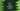# Java program to print an identity matrix### Java program to print an identity matrix :

In this tutorial, we will learn how to print one identity matrix using Java programming language. Identity matrix is a matrix whose diagonal elements are 1. Diagonal elements starting from top left to right end should be 1 and other elements are 0 of that matrix. For example, the following matrix is an identity matrix :

``````1 0 0 0 0
0 1 0 0 0
0 0 1 0 0
0 0 0 1 0
0 0 0 0 1``````

To print this matrix, we will use two for loops. One inner loop and one outer loop. If the current pointers for both loops are same, print 1, else print 0. Because for the first line 1 is on matrix position. For the second-line 1 is on matrix position etc. Let’s take a look at the program :

### Java program :

``````import java.util.Scanner;

public class Main {

public static void main(String[] args) {
//1
Scanner sc = new Scanner(System.in);
int size;

//2
System.out.println("Enter the size of the matrix :");
size = sc.nextInt();

//3
for (int i = 0; i < size; i++) {
//4
for (int j = 0; j < size; j++) {
//5
if (i == j) {
System.out.print("1 ");
} else {
System.out.print("0 ");
}
}
//6
System.out.println();
}

}

}``````

### Explanation :

The commented numbers in the above program denote the step-numbers below:

1. Create one Scanner object sc to read user-input values, integer size to store the size of the matrix.
2. Ask the user to enter the size of the matrix. Read it and save it in size variable.
3. Run one for loop. This loop will run size number of times.
4. Run one inner for loop. This loop will also run size number of times.
5. For each iteration, check if i is equal to j or not. If yes, print 1, else print 0.
6. Print one new line.

### Sample Output :

``````Enter the size of the matrix :
5
1 0 0 0 0
0 1 0 0 0
0 0 1 0 0
0 0 0 1 0
0 0 0 0 1

Enter the size of the matrix :
3
1 0 0
0 1 0
0 0 1

Enter the size of the matrix :
7
1 0 0 0 0 0 0
0 1 0 0 0 0 0
0 0 1 0 0 0 0
0 0 0 1 0 0 0
0 0 0 0 1 0 0
0 0 0 0 0 1 0
0 0 0 0 0 0 1``````